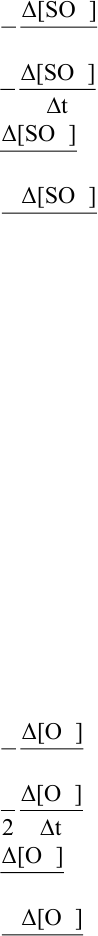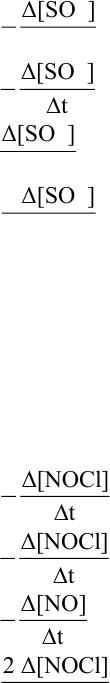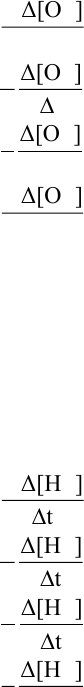Study Guides (390,000)
CA (150,000)
UTSC (10,000)
Chemistry (200)
Final

# CHMA10H3 Lecture Notes - Lecture 12: Pearson Education, Polarimeter, Chemical EquationExam

Department
Chemistry
Course Code
CHMA10H3
Professor
Ann Verner
Study Guide
Final

This preview shows pages 1-3. to view the full 36 pages of the document.1
Copyright (c) 2011 Pearson Education, Inc.
Chemistry: A Molecular Approach, 2e (Tro)
Chapter 13 Chemical Kinetics
Multiple Choice Questions
1) Given the following balanced equation, determine the rate of reaction with respect to [SO2].
2 SO2 (g) + O2(g) → 2 SO3(g)
A) Rate =
2
Δ[SO ]
1
2 Dt
B) Rate =
2
Δ[SO ]
1
2t
+
C) Rate =
2
Δ[SO ]
Dt
D) Rate =
2
2 Δ[SO ]
t
+
Diff: 2 Page Ref: 13.2
2) Identify the methods used to monitor a reaction as it occurs in the reaction flask.
A) polarimeter
B) spectrometer
C) pressure measurement
D) none of the above
E) all of the above
Diff: 1 Page Ref: 13.2
3) Given the following balanced equation, determine the rate of reaction with respect to [O2].
2 SO2 (g) + O2(g) → 2 SO3(g)
A) Rate =
2
Δ[O ]
1
2 Dt
B) Rate =
2
Δ[O ]
1
2t
+
C) Rate =
2
Δ[O ]
Dt
D) Rate =
2
2 Δ[O ]
t
+
Diff: 2 Page Ref: 13.2

Only pages 1-3 are available for preview. Some parts have been intentionally blurred.2
Copyright (c) 2011 Pearson Education, Inc.
4) Given the following balanced equation, determine the rate of reaction with respect to [SO3].
2 SO2 (g) + O2(g) → 2 SO3(g)
A) Rate =
3
Δ[SO ]
1
2 Dt
B) Rate =
3
Δ[SO ]
1
2t
+
C) Rate =
3
Δ[SO ]
Dt
D) Rate =
3
2 Δ[SO ]
t
+
Diff: 2 Page Ref: 13.2
5) Given the following balanced equation, determine the rate of reaction with respect to [NOCl].
2 NO(g) + Cl2(g) → 2 NOCl(g)
A) Rate =
1Δ[NOCl]
2t
B) Rate =
1Δ[NOCl]
2t
+
C) Rate =
D) Rate =
2 Δ[NOCl]
t
Diff: 2 Page Ref: 13.2

Only pages 1-3 are available for preview. Some parts have been intentionally blurred.3
Copyright (c) 2011 Pearson Education, Inc.
6) Given the following balanced equation, determine the rate of reaction with respect to [O2].
2 O3(g) → 3 O2(g)
A) Rate =
2
2 Δ[O ]
t
B) Rate =
2
Δ[O ]
2
3t
C) Rate =
2
Δ[O ]
1
3 Dt
+
D) Rate =
2
3 Δ[O ]
t
+
Diff: 2 Page Ref: 13.2
7) Given the following balanced equation, determine the rate of reaction with respect to [H2].
N2(g) + 3 H2(g) → 2 NH3(g)
A) Rate =
2
3 Δ[H ]
t
+
B) Rate =
2
Δ[H ]
2
3t
C) Rate =
2
Δ[H ]
3
2t
+
D) Rate =
2
Δ[H ]
1
3t